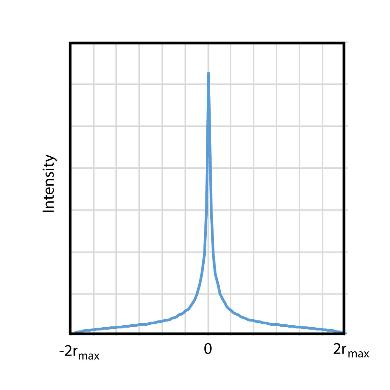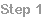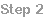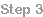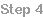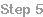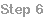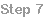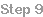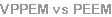Step 7
Our basic explanation is split into several stepsPage 3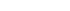VPPEM spatial resolution
The spatial resolution is determined by the maximum cyclotron
radius of the electrons as they leave the sample surface. The
radius depends on the energy of the electrons and the strength of
the magnetic field at the surface.
A disk of confusion is created by electrons being emitted at all
angles up to 90o from the magnetic axis.

The maximum off-axis distance is twice the maximum radius. The
helical trajectories have a maximum radius that depend on the
energy of the electrons E, and the magnetic field B, in the following
relationship: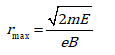The probability of being at a distance x from the point of emission
can be calculated using this geometry: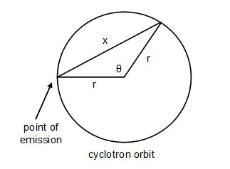The cyclotron orbit is illustrated by the circle of radius r.  The
point the electron crosses the image plane will be a distance x
from the point of emission where x is given by the cosine law:
For a uniform distribution of radii from 0 to xmax, t:he probability
of an electron crossing the image plane between x and  x+dx  is: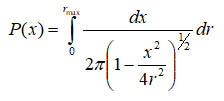This equation can be integrated numerically. This gives the one
dimensional PSF. Dividing the result by 2πr,  the area of the PSF
at a radius r, gives the cross section of the 2 dimensional PSF as
shown on the right.

While the one dimensional PSF is sharply peaked at the center,
nearly 50% of the integrated intensity is at a radius greater than 1
rmax.
VPPEM Point Spread Function (PSF)
edge resolution in microns approximately equals:

with E in electron volts and B  in Tesla.

While the energy of the electrons can be changed within the field
by biasing the sample, but this does not change the spatial
resolution. The angle of deflection due to terminating the vector
potential field is inversely proportional to the square root of the
energy of the electrons when they exit the field.   However, the
angles of the cyclotron orbits also change in the same way as
the electrons are accelerated or decelerated.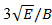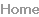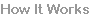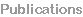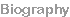How it works